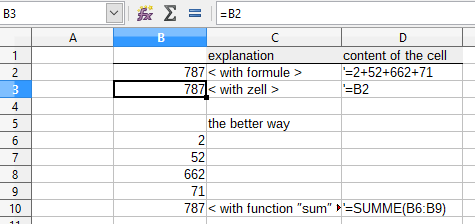# How to count number of operation?

What is the formule to count the number of operation in Calc ? like in one cell i write this “=2+52+662+71” And need the answer in other cell “4”. Thanks so much for your help

You can do cell linking, e.g. “= B2” work.Or you make a simple summation. Set the cursor e.g. in cell B10 and click the sums icon.
See attached file.

Lösung für Summe.ods

Hello ! Danke for the fast answer !
I just dont be enough clear. What i need is the number of “calcul”.
For example :
1+2+3+4+5+6 = 6 or 7+8 = 2 or 55+21+41+18=4

(I’ve search on internet, and the closest answer was trough Excel with the extension TEXTEFORMULE of MoreFunC)

Oh sorry, i misunderstood. There is no corresponding function in Calc itself. I did not find it.
Have you already looked at the existing extensions?

https://extensions.libreoffice.org/

Quoting @Sergent:
“What is the formula to count the number of operations in Calc? Like in one cell I write this “=2+52+662+71” And need the answer in other cell “4”. …”

The supposed result “4” for your example does not count the number of operations but the numbert of operands. The calculation explicitly is demanding 3 operations. How Calc does it in the background, and how many operations are needed then we don’t know…

What result would you expect if the formula was `=IF(A1+A2<100;2+52*662+71;0)`?

To get a formula contained in a cell as a text result you have the function `FORMULA()` in Calc. (I didn’t check for its French name. Suppose it’s `TEXTEFORMULE`.)

To count how many cells in a range, say `A1:A6`, contain a formula you can use
`{=COUNTIF(NOT(ISERROR(FORMULA(A1:A6)));TRUE())}` entered for array evaluation with Ctrl+Shift+Enter.
The much simpler `{=COUNTIF(ISFORMULA(A1:A6);TRUE())}` or `=SUMPRODUCT(ISFORMULA(A1:A6))` should also work, but there is a bug. A fix was recently announced. It shall be available in V 6.1.1.

For accumulating functions used on simple ranges like `SUM(A1:C6)` you can use
`=COLUMNS(A1:C6)*ROWS(A1:C6)-SUMPRODUCT(NOT(ISNUMBER(A1:C6)))` in an additional cell to get the number of (actual = numeric) operands. The overall size of the range is simply `=COLUMNS(A1:C6)*ROWS(A1:C6)`.

Back to the simple continued sums like in the original example:
For `=1+2+3+4+A4+99` in cell `S1` `=LEN(S1)-LEN(SUBSTITUTE(S1;"+";""))` would return the number of adding operands ("+") =5 and an additional +1 appended to the formula would produce the 6 as the number of operands (addends) including single references. To do similar things for expressions containing different operands and/or parentheses or… it’s much more complicated.

Editing1:
Sorry! The last formula assumed S1 to contain the formula as a text.
S1 being the cell where the adding formula was entered the operand-counting formula must be:
`=LEN(FORMULA(S1))-LEN(SUBSTITUTE(FORMULA(S1);"+";""))`

Thanks a lot for this complet answer !!
The last one is exactly what i need :
`=LEN(S1)-LEN(SUBSTITUTE(S1;"+";""))`
… unfortunately in my case it return : `#NAME?`
Any suggestion ?
(Format rectified by @Lupp .)

Set your UI language to some English, or at least set
Tools>Options>LibreOffice Calc>Formula>Use English function names enabled.
The other option is to guess in a clever way, probably searching through the list of availebla functions in the formula wizard.
(To use common terms is a basic requirement for the usefulness of international forums. Don’t be stubborn concerning your local language… I’m a German living in Germany. So is @Hrbrgr .)

Woaw ! It works now using your formula : =LEN(FORMULA(S1))-LEN(SUBSTITUTE(FORMULA(S1);"+";"")). Thanks a loooot !!
I needed to add +1 to the formula to get the correct number i wanted.
Now i’m in front of a new issue : I’ve some cell with just one number (without +) so it display #N/A and ruined my total…
Any idea how to consider this #N/A as 0 ?

Just a hint: To find the cause of a problem and subsequently a solution yourself now and then is more fun.
The formula I suggested uses the Calc function FORMULA(). It cannot work on Cell content that is not a formula at all. If there is a single number, say 13, but made up to be returned as a formula result by entering =13 the #N/A will vanish.
If you want to also analyse expressions given as texts in the place of formulae lead by the “=”, things get a bit more complicated again.

Thanks you so much for your assistance et rapidity ! Thank you, Merci !!! I’ve to make the topic as “Solved” if i find how…!